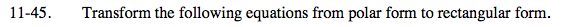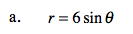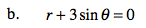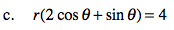Home > PC > Chapter 11 > Lesson 11.1.3 > Problem11-45

11-45.
1. Transform the following equations from polar form to rectangular form. Homework Help ✎

1. r = 6 sin θ

2. r + 3 sin θ = 0

3. r(2 cos θ + sin θ) = 4In order to transform the equation, you need r2 and r sin(θ).
Multiply both sides of the equation by r.